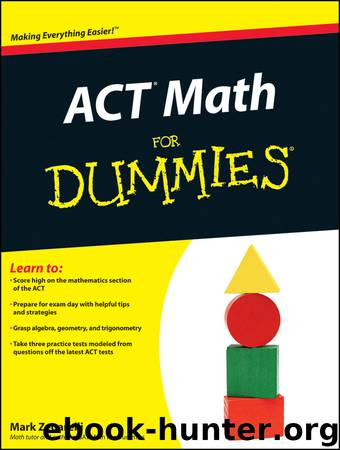# ACT Math For Dummies by Zegarelli MarkAuthor:Zegarelli, Mark [Zegarelli, Mark]
Language: eng
Format: epub, mobi
Tags: Itzy, Kickass.so
ISBN: 9781118001547
Amazon: B005CB2UV4
Publisher: For Dummies
Published: 2011-06-09T04:00:00+00:00

When you know both the slope (m = 2) and the y-intercept (b = –4), you can write the equation in slope-intercept form:

So the correct answer is Choice (G).

You also can use the slope-intercept form to answer trickier questions (I told you this form would come in handy). Use the information provided in the problem to find the equation of the line, and then answer the question. The following example walks you through a question like this one.

Which of the following is the equation of a line that includes the point (10, –1) and has a slope of ?

(A) –x + 10y = 3

(B) 10x – y = 5

(C) 3x + 5y = 9

(D) 3x – 5y = 35

(E) –3x + 5y = 7

To answer this question, you want to use the slope-intercept form, so you need to know the values of m and b. You’re given the slope, so m = . To find the value of b, plug in the information from the point that’s given (x = 10 and y = –1), and then simplify and solve for b: